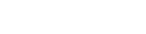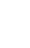# JavaScript Loop

In JavaScript, loops are used to conduct repetitive activities based on a condition.

In JavaScript, there are four different forms of loops.

• for loop
• while loop
• do-while loop
• for-in loop

## for loop

For loops are typically used to repeat code for a certain number of times.

``````
for (initialization; condition; finalExpression) {
// code block
}

``````

### Lets take an example and iterate through integers from 1-5

``````
for (i=1; i<=5; i++)  {
document.write(i + "<br/>")
}

``````
##### Output
``````
1
2
3
4
5

``````

## while loop

The while loop iterates across a block of code as long as a condition is met.

``````
while (condition)
{
// code block
}

``````

### Lets use an example where we cycle through the integers from 1 to 5.

``````
let i = 1;
while (i <= 5) {
document.write(i + "<br/>")
i++;
}

``````
##### Output
``````
1
2
3
4
5

``````

## do-while loop

Whether the condition is true or not, the do while loop script is executed at least once.

``````
do{
// code block
} while (condition);

``````

### Lets take an example and iterate over the integers 1 to 5

``````
var i=1;
do{
document.write(i + "<br/>");
i++;
}while (i<=5);

``````
##### Output
``````
1
2
3
4
5

``````

## for-in loop

The JavaScript for in statement iterates across an Objects properties.

``````
for (key in object) {
// code block
}

``````

### Lets take an example and iterate through Objects properties.

``````
const employee = {emiId:"101", empTitle:"Developer", age:36};

let result = "";
for (let val in employee) {
result += employee[val] + " ";
}

``````
##### Output
``````
101 Developer 36

``````